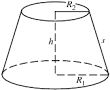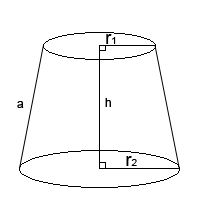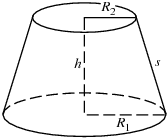## How to Calculate and Solve for the Height and Volume of a Conical Frustum | The Calculator EncyclopediaThe image above is a conical frustum.

To compute the volume of a conical frustum, three essential parameters are needed and this parameters are radius of the lower base (R), radius of the upper base (r) and height (h).

The formula for calculating the volume of a conical frustum:

V = πh(R² + Rr + r²)3

Where;
V = Volume of the conical frustum
R = Radius of the lower base
r = Radius of the upper base
h = Height of the conical frustum

Let’s solve an example;
Find the volume of the conical frustum when the lower base is 5 cm with an upper base of 9 cm and a height of 11 cm.

This implies that;
R = Radius of the lower base = 5 cm
r = Radius of the upper base = 9 cm
h = Height of the conical frustum = 11 cm

V = π(11)((5)² + (5)(9) + (9)²)3
V = (34.557)((25) + (45) + (81))3
V = (34.557)(151)3
V = 5218.1853
V = 1739.39

Therefore, the volume of the conical frustum is 1739.39 cm3.

## How to Calculate and Solve for the Total Surface Area of a Conical Frustum | The Calculator EncyclopediaThe image above is a conical frustum.

To compute the total surface area of a conical frustum requires three essential parameters which are the radius of the lower base (R), radius of the upper base (r) and the height (h).

The formula for calculating the total surface area of a conical frustum;

A = π[R² + r² + (R + r)√((R – r)² + h²)]

Where;
A = Total surface area of the conical frustum
R = Radius of the lower base
r = Radius of the upper base
h = Height of the conical frustum

Let’s solve an example;
Find the total surface area of the conical frustum when the radius of the upper base is 11 cm, radius of the lower base is 17 cm and the height is 30 cm.

This implies that;
r = Radius of the upper base = 11 cm
R = Radius of the lower base = 17 cm
h = Height of the conical frustum = 30 cm

A = π[R² + r² + (R + r)√((R – r)² + h²)]
A = π[17² + 11² + (17 + 11)√((17 – 11)² + 30²)]
A = π[289 + 121 + (28)√((6)² + 900)]
A = π[289 + 121 + (28)√(36+ 900)]
A = π[289 + 121 + (28)√(936)]
A = π[289 + 121 + (28)(30.59)]
A = π[289 + 121 + 856.63]
A = π[1266.63]
A = 3979.25

Therefore, the total surface area of the conical frustum is 3979.25 cm².

## How to Calculate and Solve for the Lateral Surface Area of a Conical Frustum | Nickzom CalculatorThe image above is a conical frustum.

To compute the lateral surface area of a conical frustum requires three essential parameters which are the radius of the lower base (R), radius of the upper base (r) and the height (h).

The formula for calculating the lateral surface area of a conical frustum:

A = π(R + r)√((R – r)² + h²)

Where;
A = Area of the conical frustum
R = Radius of the lower base
r = Radius of the upper base
h = Height of the conical frustum

Let’s solve an example;
Given that the height of a conical frustum is 28 cm with a radius of lower base of 22 cm and a radius of upper base of 19 cm. Find the lateral surface area of the conical frustum?

This implies that;
h = Height of the conical frustum = 28 cm
R = Radius of the lower base = 22 cm
r = Radius of the upper base = 19 cm

A = π(R + r)√((R – r)² + h²)
A = 3.142(22 + 19)√((22 – 19)² + 28²)
A = 3.142(41)√((3)² + 28²)
A = 3.142 (41)√(9 + 784)
A = 3.142 (41)√(793)
A = 3.142 (41)(28.16)
A = 3.142 x 1154.56
A = 3627.63

Therefore, the lateral surface area of the conical frustum is 3627.63 cm².